## 3.6Overview of discretisation

The choice of numerical method determines how coeﬃcients for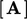and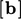are calculated and consequently the characteristics of the resulting matrix equation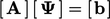.

The ﬁnite volume method described here is ﬁrmly rooted in the underlying concept of control volumes, described in Sec. 3.1 . It uses integrals over a surface surrounding a volume applied to irregular polyhedral meshes, described in Sec. 3.2 .

The discretisation is described in terms of diﬀerential operators, e.g.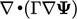and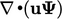, applied to a general ﬁeld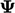.4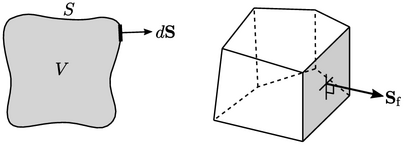The main concept is that faces within a mesh form closed surfaces surrounding ﬁnite volumes, e.g. a single cell. Any surface integral that represents a derivative, e.g.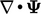, is approximated by a summation over the faces ‘’ that form the surface, i.e.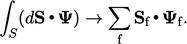The ﬂux associated with, i.e.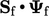, must then be calculated. The value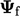is required at each face, which must be calculated by some method of interpolation of values of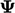from cells neighbouring the respective face.

### Intensive and extensive properties

In this chapter, derivatives and their discretisation are described at a point, e.g.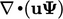, e.g. Eq. (3.8 ):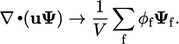Calculating a derivative with this expression using the known ﬁeldsimply produces another ﬁeld (with values deﬁned at cell centres, with units of/time).

The resulting ﬁeld is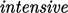, meaning it is independent of the size of the system/geometry. Like other intensive ﬁelds, e.g.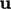andthemselves, it can be used in further calculations, e.g. within another derivative or added/subtracted from other ﬁelds.

Extensive properties are dependent on the size of the system. For example, the volumetric ﬂux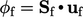described in Sec. 3.9 is dependent on the face areas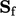. Numerical operations involving extensive properties, e.g. addition, subtraction, or mapping to another location, generally produce meaningless data.

While calculations of derivatives yield intensive ﬁelds, matrix equations are constructed in extensive form, with coeﬃcients and source vector scaled by cell volume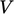. In other words, in the discretisation example above, the multiplication by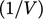would be omitted.

There is no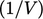multiplier in the discretisation of terms which do not involve a surface integral, e.g. time derivative Eq. (3.21 ) and terms in Sec. 3.20 . For those terms, the calculation of matrix coeﬃcients and sources includes a multiplication by.

4consistent with the idea of “Field Operation and Manipulation”, which forms the acronym “FOAM” (later, OpenFOAM) used for the CFD software created by author Henry Weller.

Notes on CFD: General Principles - 3.6 Overview of discretisation